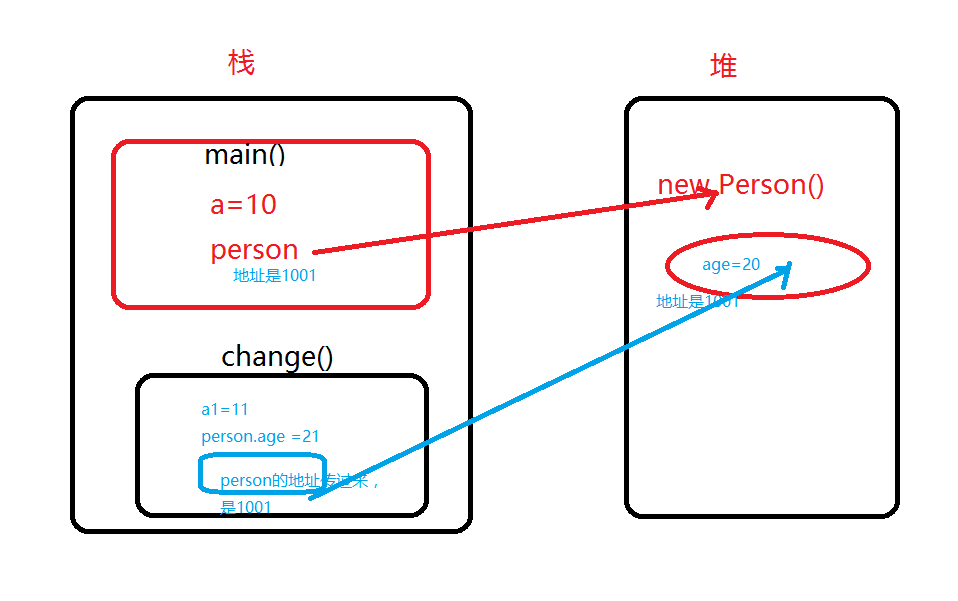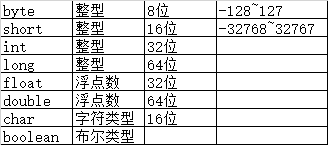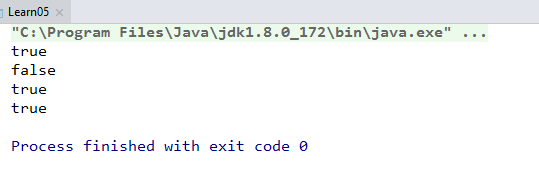﻿ 简单谈谈Java中的栈和堆_java_脚本之家
java# 简单谈谈Java中的栈和堆

Java的栈中存储以下类型数据，栈对应的英文单词是StackJava的堆中存储以下类型数据，堆对应的英文单词是Heap```class Person {

int age;

}

public class LearnHeap {

public static void main(String args[]){

int a=10;
Person person = new Person();
person.age =20;

change(a,person);
System.out.println("a="+ a+",and person.age = "+person.age);

}

static void change(int a1, Person person){

a1 = 11;
person.age= 21;
System.out.println("a1="+ a1+",and age1 = "+person);

}

}``````package heapandStack;

public class LearnHeap02 {

public static void main(String args[]){

byte b=10;
short s=20;
int i=30;
long l =40l;

float f =12.34f;
double d = 100.123d;

char c = 'A';
boolean flag = true;

change(b,s,i,l,f,d,c,flag);

System.out.println(b);
System.out.println(s);
System.out.println(i);
System.out.println(l);

System.out.println(f);
System.out.println(d);
System.out.println(c);
System.out.println(flag);

}

static void change(byte a, short b, int c,long d, float f, double g, char h,boolean x){

a =11;
b=21;
c =31;
d =41l;
f=12.99f;
g= 200.123d;
h ='V';
x =false;

System.out.println(a);
System.out.println(b);
System.out.println(c);
System.out.println(d);

System.out.println(f);
System.out.println(g);
System.out.println(h);
System.out.println(x);

}
}```

```public class LearnHeap03 {

public static void main(String args[]){

int a[] ={1,2,3};
change(a);
for(int i:a)
System.out.print(i+" ");

}

static void change(int[] a){
a=4;
a=5;
for(int i:a)
System.out.print(i+" ");
System.out.println("============ ");

}
}```Java种8种基本数据类型，并不包含String，String的值会被change函数改变吗？String应该存在栈中，还是堆中呢？

```public class Learn04 {

public static void main(String args[]){

String s1 = new String("abcd");
String s2 = "asdfghjkl";
System.out.println(s1+", "+s2);
change(s1,s2);
System.out.println(s1+", "+s2);

}

static void change(String s1,String s2){
s1 ="123456";
s2 ="000000";
System.out.println(s1+", "+s2);

}
}``````public class Learn05 {

public static void main(String args[]){

String s1 = new String("abcd");
String s2 = "abcd";
boolean a =s1.equals(s2);
boolean b =(s1==s2);

System.out.println(a);
System.out.println(b);

String s3 = "abcd";
boolean a1 =s3.equals(s2);
boolean b1 =(s3==s2);

System.out.println(a1);
System.out.println(b1);

}

}```